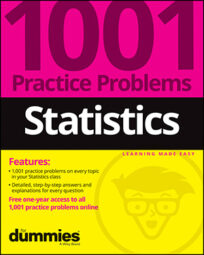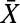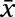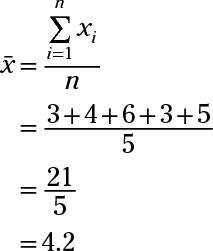##### Statistics: 1001 Practice Problems For Dummies (+ Free Online Practice)The sample questions here focus on working with the sample means as a random variable. You have a six-sided die with faces numbered 1 through 6, and each face is equally likely to come up on any given roll.

## Sample questions

1. What is the meaning for X in this example?

Answer: a random variable denoting the outcome from a single roll of the die

X is a random variable with possible values 1, 2, 3, 4, 5, and 6, denoting the outcome from a single roll of the die.

2. What is the meaning of the following in this example?Answer: a random variable denoting the average value when you roll the die n times (where n is some fixed number)is a random variable representing any calculated average from a certain number of rolls of the die.

3. Suppose that you rolled the die five times and got the values of 3, 4, 6, 3, and 5. What is the value of the following?represents the sample mean; you find it by adding the numbers and dividing by n (the sample size). Use the following formula:Here, each xi represents a value in the data set — x1 is the first number, x2 is the second number, and so on, and then xn is the nth, or last, number.

If you need more practice on this and other topics from your statistics course, visit 1,001 Statistics Practice Problems For Dummies to purchase online access to 1,001 statistics practice problems! We can help you track your performance, see where you need to study, and create customized problem sets to master your stats skills.